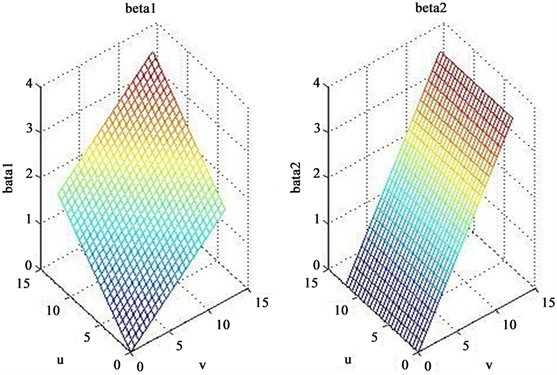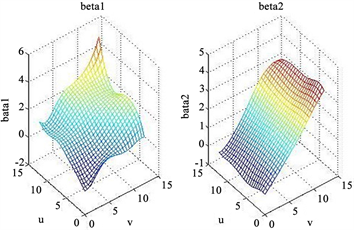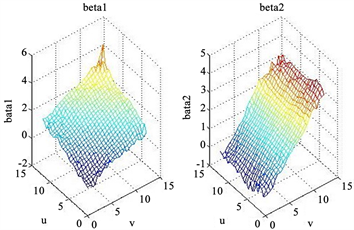#### 期刊菜单

Local Nonlinear BGWR Estimation of Spatially Varying Coefficient Model
DOI: 10.12677/AAM.2018.710144, PDF, HTML, XML, 下载: 695  浏览: 3,402

Abstract: Based on the local nonlinear GWR fitting method of the spatial variable coefficient model, the local nonlinear BGWR fitting method of the spatial variable coefficient model is given. Estimates of model parameters at various geographic locations are obtained by Gibbs sampling. At the same time, the simulation experiment is designed. By comparing the method with the local nonlinear GWR estimation method, the accuracy of the local nonlinear BGWR method is shown.

1. 引言

Brunsdon等  首次明确提出了空间变系数模型，同时给出了该模型的地理加权回归(geographical weighed regression，简称GWR)方法，即以观测点之间的距离函数为权值的非参数光滑估计方法。Wang Ning等   将变系数模型的局部线性拟合方法推广到空间变系数模型的情况，给出了局部线性GWR方法。程鹏鹏  提出了局部非线性GWR拟合方法，在降低系数函数估计的偏和边界效应上较局部线性GWR方法更显著。LeSage   提出了贝叶斯地理加权回归(Bayesian geographical weighed regression，简称BGWR)模型，首先运用Gibbs抽样技术给出一组参数用来修正异常观测值，由贝叶斯公式得到待估参数的条件后验概率  ，保证估计结果的稳健性，之后再根据GWR方法的原理进行估计。冯金杰  通过空间加权距离构造权重矩阵，基于多元线性回归模型的贝叶斯统计推断  ，得到了该模型的局部线性BGWR估计方法。

2. 空间变系数模型的局部非线性BGWR估计

${Y}_{i}=\underset{j=1}{\overset{p}{\sum }}{\beta }_{j}\left({u}_{i},{v}_{i}\right){X}_{ij}+{\epsilon }_{i},\text{\hspace{0.17em}}\text{\hspace{0.17em}}\text{\hspace{0.17em}}i=1,2,\cdots ,n$ (1)

$\begin{array}{l}{\beta }_{j}\left(u,v\right)\approx {\beta }_{j}\left({u}_{0},{v}_{0}\right)+{\beta }_{j}^{\left(u\right)}\left({u}_{0},{v}_{0}\right)\left(u-{u}_{0}\right)+{\beta }_{j}^{\left(v\right)}\left({u}_{0},{v}_{0}\right)\left(v-{v}_{0}\right)+\frac{1}{2!}{\beta }_{j}^{\left(uu\right)}\left({u}_{0},{v}_{0}\right){\left(u-{u}_{0}\right)}^{2}\\ \text{\hspace{0.17em}}\text{\hspace{0.17em}}\text{\hspace{0.17em}}\text{\hspace{0.17em}}\text{\hspace{0.17em}}\text{\hspace{0.17em}}\text{\hspace{0.17em}}\text{\hspace{0.17em}}\text{\hspace{0.17em}}\text{\hspace{0.17em}}\text{\hspace{0.17em}}\text{\hspace{0.17em}}\text{\hspace{0.17em}}\text{\hspace{0.17em}}\text{ }\text{ }+\frac{1}{2!}{\beta }_{j}^{\left(vv\right)}\left({u}_{0},{v}_{0}\right){\left(v-{v}_{0}\right)}^{2}+\frac{1}{2!}{\beta }_{j}^{\left(uv\right)}\left({u}_{0},{v}_{0}\right)\left(u-{u}_{0}\right)\left(v-{v}_{0}\right)\end{array}$ (2)

$\begin{array}{l}\underset{i=1}{\overset{n}{\sum }}\left[{Y}_{i}-\underset{j=1}{\overset{p}{\sum }}\left({\beta }_{j}\left({u}_{0},{v}_{0}\right)+{\beta }_{j}^{\left(u\right)}\left({u}_{0},{v}_{0}\right)\left(u-{u}_{0}\right)+{\beta }_{j}^{\left(v\right)}\left({u}_{0},{v}_{0}\right)\left(v-{v}_{0}\right)+\frac{1}{2!}{\beta }_{j}^{\left(uu\right)}\left({u}_{0},{v}_{0}\right){\left(u-{u}_{0}\right)}^{2}\\ +\frac{1}{2!}{\beta }_{j}^{\left(vv\right)}\left({u}_{0},{v}_{0}\right){\left(v-{v}_{0}\right)}^{2}+{\beta }_{j}^{\left(uv\right)}\left({u}_{0},{v}_{0}\right)\left(u-{u}_{0}\right)\left(v-{v}_{0}\right)\right){{X}_{ij}\right]}^{2}{K}_{h}\left({d}_{0i}\right)\end{array}$ (3)

${K}_{h}\left({d}_{0i}\right)=K\left(\frac{{d}_{0i}}{h}\right)={\omega }_{i}\left({u}_{0},{v}_{0}\right)\text{\hspace{0.17em}}\text{\hspace{0.17em}}\left(i=1,2,\cdots ,n\right)$

$W\left({u}_{0},{v}_{0}\right)=Diag\left({\omega }_{1}\left({u}_{0},{v}_{0}\right),{\omega }_{2}\left({u}_{0},{v}_{0}\right),\cdots ,{\omega }_{n}\left({u}_{0},{v}_{0}\right)\right)$

$\begin{array}{c}P\left({u}_{0},{v}_{0}\right)=\left[{\beta }_{1}\left({u}_{0},{v}_{0}\right),\cdots ,{\beta }_{p}\left({u}_{0},{v}_{0}\right),{\beta }_{1}^{\left(u\right)}\left({u}_{0},{v}_{0}\right),\cdots ,{\beta }_{p}^{\left(u\right)}\left({u}_{0},{v}_{0}\right),\\ \text{\hspace{0.17em}}\text{\hspace{0.17em}}\text{ }\text{ }\text{ }{\beta }_{1}^{\left(v\right)}\left({u}_{0},{v}_{0}\right),\cdots ,{\beta }_{p}^{\left(v\right)}\left({u}_{0},{v}_{0}\right),\cdots ,{\beta }_{1}^{\left(uu\right)}\left({u}_{0},{v}_{0}\right),\cdots ,{\beta }_{p}^{\left(uu\right)}\left({u}_{0},{v}_{0}\right),\\ \text{ }\text{\hspace{0.17em}}\text{\hspace{0.17em}}\text{ }\text{ }{\beta }_{1}^{\left(vv\right)}\left({u}_{0},{v}_{0}\right),\cdots ,{\beta }_{p}^{\left(vv\right)}\left({u}_{0},{v}_{0}\right),{\beta }_{1}^{\left(uv\right)}\left({u}_{0},{v}_{0}\right),\cdots ,{{\beta }_{p}^{\left(uv\right)}\left({u}_{0},{v}_{0}\right)\right]}^{\text{T}}\end{array}$

${A}_{1}=\left(\begin{array}{cccc}{X}_{11}& \cdots & {X}_{1,p-1}& {X}_{1p}\\ {X}_{21}& \cdots & {X}_{2,p-1}& {X}_{2p}\\ ⋮& & ⋮& ⋮\\ {X}_{n1}& \cdots & {X}_{n,p-1}& {X}_{np}\end{array}\right)$

${A}_{2}=\left(\begin{array}{cccc}{X}_{11}\left({u}_{1}-{u}_{0}\right)& \cdots & {X}_{1,p-1}\left({u}_{1}-{u}_{0}\right)& {X}_{1p}\left({u}_{1}-{u}_{0}\right)\\ {X}_{21}\left({u}_{2}-{u}_{0}\right)& \cdots & {X}_{2,p-1}\left({u}_{2}-{u}_{0}\right)& {X}_{2p}\left({u}_{2}-{u}_{0}\right)\\ ⋮& & ⋮& ⋮\\ {X}_{n1}\left({u}_{n}-{u}_{0}\right)& \cdots & {X}_{n,p-1}\left({u}_{n}-{u}_{0}\right)& {X}_{np}\left({u}_{n}-{u}_{0}\right)\end{array}\text{\hspace{0.17em}}\text{\hspace{0.17em}}\begin{array}{cccc}{X}_{11}\left({v}_{1}-{v}_{0}\right)& \cdots & {X}_{1,p-1}\left({v}_{1}-{v}_{0}\right)& {X}_{1p}\left({v}_{1}-{v}_{0}\right)\\ {X}_{21}\left({v}_{2}-{v}_{0}\right)& \cdots & {X}_{2,p-1}\left({v}_{2}-{v}_{0}\right)& {X}_{2p}\left({v}_{2}-{v}_{0}\right)\\ ⋮& & ⋮& ⋮\\ {X}_{n1}\left({v}_{n}-{v}_{0}\right)& \cdots & {X}_{n,p-1}\left({v}_{n}-{v}_{0}\right)& {X}_{np}\left({v}_{n}-{v}_{0}\right)\end{array}\right)$

${A}_{3}=\left(\begin{array}{cccc}{X}_{11}{\left({u}_{1}-{u}_{0}\right)}^{2}& \cdots & {X}_{1,p-1}{\left({u}_{1}-{u}_{0}\right)}^{2}& {X}_{1p}{\left({u}_{1}-{u}_{0}\right)}^{2}\\ {X}_{21}{\left({u}_{2}-{u}_{0}\right)}^{2}& \cdots & {X}_{2,p-1}{\left({u}_{2}-{u}_{0}\right)}^{2}& {X}_{2p}{\left({u}_{2}-{u}_{0}\right)}^{2}\\ ⋮& & ⋮& ⋮\\ {X}_{n1}{\left({u}_{n}-{u}_{0}\right)}^{2}& \cdots & {X}_{n,p-1}{\left({u}_{n}-{u}_{0}\right)}^{2}& {X}_{np}{\left({u}_{n}-{u}_{0}\right)}^{2}\end{array}\begin{array}{cccc}{X}_{11}{\left({v}_{1}-{v}_{0}\right)}^{2}& \cdots & {X}_{1,p-1}{\left({v}_{1}-{v}_{0}\right)}^{2}& {X}_{1p}{\left({v}_{1}-{v}_{0}\right)}^{2}\\ {X}_{21}{\left({v}_{2}-{v}_{0}\right)}^{2}& \cdots & {X}_{2,p-1}{\left({v}_{2}-{v}_{0}\right)}^{2}& {X}_{2p}{\left({v}_{2}-{v}_{0}\right)}^{2}\\ ⋮& & ⋮& ⋮\\ {X}_{n1}{\left({v}_{n}-{v}_{0}\right)}^{2}& \cdots & {X}_{n,p-1}{\left({v}_{n}-{v}_{0}\right)}^{2}& {X}_{np}{\left({v}_{n}-{v}_{0}\right)}^{2}\end{array}\right)$

${A}_{4}=\left(\begin{array}{cccc}{X}_{11}\left({u}_{1}-{u}_{0}\right)\left({v}_{1}-{v}_{0}\right)& \cdots & {X}_{1,p-1}\left({u}_{1}-{u}_{0}\right)\left({v}_{1}-{v}_{0}\right)& {X}_{1p}\left({u}_{1}-{u}_{0}\right)\left({v}_{1}-{v}_{0}\right)\\ {X}_{21}\left({u}_{2}-{u}_{0}\right)\left({v}_{2}-{v}_{0}\right)& \cdots & {X}_{2,p-1}\left({u}_{2}-{u}_{0}\right)\left({v}_{2}-{v}_{0}\right)& {X}_{2p}\left({u}_{2}-{u}_{0}\right)\left({v}_{2}-{v}_{0}\right)\\ ⋮& & ⋮& ⋮\\ {X}_{n1}\left({u}_{n}-{u}_{0}\right)\left({v}_{n}-{v}_{0}\right)& \cdots & {X}_{n,p-1}\left({u}_{n}-{u}_{0}\right)\left({v}_{n}-{v}_{0}\right)& {X}_{np}\left({u}_{n}-{u}_{0}\right)\left({v}_{n}-{v}_{0}\right)\end{array}\right)$

$\stackrel{^}{B}\left({u}_{0},{v}_{0}\right)={\left[{X}^{\text{T}}\left({u}_{0},{v}_{0}\right)W\left({u}_{0},{v}_{0}\right)X\left({u}_{0},{v}_{0}\right)\right]}^{-1}{X}^{\text{T}}\left({u}_{0},{v}_{0}\right)W\left({u}_{0},{v}_{0}\right)Y$ (4)

$\begin{array}{c}L\left[B\left({u}_{0},{v}_{0}\right),\sigma \right]={\left(\frac{1}{2\pi {\sigma }^{2}}\right)}^{\frac{n}{2}}\mathrm{exp}\left\{-\frac{1}{2{\sigma }^{2}}{\left[Y-X\left({u}_{0},{v}_{0}\right)B\left({u}_{0},{v}_{0}\right)\right]}^{\text{T}}\left[Y-X\left({u}_{0},{v}_{0}\right)B\left({u}_{0},{v}_{0}\right)\right]\right\}\\ ={\left(\frac{1}{2\pi {\sigma }^{2}}\right)}^{\frac{n}{2}}\mathrm{exp}\left\{-\frac{1}{2{\sigma }^{2}}\left[{\left[Y-X\left({u}_{0},{v}_{0}\right)\stackrel{^}{B}\left({u}_{0},{v}_{0}\right)\right]}^{\text{T}}\left[Y-X\left({u}_{0},{v}_{0}\right)\stackrel{^}{B}\left({u}_{0},{v}_{0}\right)\right]\\ \underset{}{\overset{}{}}+{\left[B\left({u}_{0},{v}_{0}\right)-\stackrel{^}{B}\left({u}_{0},{v}_{0}\right)\right]}^{\text{T}}{X}^{\text{T}}\left({u}_{0},{v}_{0}\right)X\left({u}_{0},{v}_{0}\right)\left[B\left({u}_{0},{v}_{0}\right)-\stackrel{^}{B}\left({u}_{0},{v}_{0}\right)\right]\right]\right\}\\ ={\left(\frac{1}{2\pi {\sigma }^{2}}\right)}^{\frac{n}{2}}\mathrm{exp}\left\{-\frac{1}{2{\sigma }^{2}}\left[{Z}_{n}^{2}\left(n-6p\right)+{\left[B\left({u}_{0},{v}_{0}\right)-\stackrel{^}{B}\left({u}_{0},{v}_{0}\right)\right]}^{\text{T}}\\ \underset{}{\overset{}{}}\cdot {X}^{\text{T}}\left({u}_{0},{v}_{0}\right)X\left({u}_{0},{v}_{0}\right)\left[B\left({u}_{0},{v}_{0}\right)-\stackrel{^}{B}\left({u}_{0},{v}_{0}\right)\right]\right]\right\}\end{array}$

$\begin{array}{c}\pi \left(\beta \left({u}_{0},{v}_{0}\right)|Y,X\right)\propto L\left(\beta \left({u}_{0},{v}_{0}\right),\sigma |Y,X\right)\pi \left(\beta \left({u}_{0},{v}_{0}\right),\sigma \right)\\ \propto {M}_{{t}_{3p}}\left(v,\stackrel{^}{\beta }\left({u}_{0},{v}_{0}\right),{Q}_{0}\right)\end{array}$ (6)

${Z}_{n}^{2}={\left[Y-X\left({u}_{0},{v}_{0}\right)\stackrel{^}{\beta }\left({u}_{0},{v}_{0}\right)\right]}^{\text{T}}\left[Y-X\left({u}_{0},{v}_{0}\right)\stackrel{^}{\beta }\left({u}_{0},{v}_{0}\right)\right]/\left(n-6p\right).$

$\pi \left({\sigma }^{2}|Y,X\right)\propto \frac{1}{{\sigma }^{n+2}}\mathrm{exp}\left\{-\frac{\left(n-6p\right){Z}_{n}^{2}}{2{\sigma }^{2}}\right\}\propto IGamma\left(n+1,v{Z}_{n}^{2}/2\right)$ (7)

3. 模拟试验

$\left({u}_{i},{v}_{i}\right)=\left(0.5\mathrm{mod}\left(\frac{i-1}{25}\right),0.5\mathrm{int}\left(\frac{i-1}{25}\right)\right),i=1,2,\cdots ,n$

${Y}_{i}={\beta }_{1}\left({u}_{i},{v}_{i}\right)+{\beta }_{2}\left({u}_{i},{v}_{i}\right){X}_{i}+{\epsilon }_{i},\text{\hspace{0.17em}}\text{\hspace{0.17em}}i=1,2,\cdots ,n$

${\beta }_{1}\left(u,v\right)=\frac{1}{6}\left(u+v\right),\text{\hspace{0.17em}}\text{\hspace{0.17em}}{\beta }_{2}\left(u,v\right)=\frac{1}{3}u$Figure 1. Surface map of the initial values of each coefficient function(a) (b)

Figure 2. (a) Estimation of each coefficient function of the local nonlinear GWR fitting method, (b) Estimation of each coefficient function of the local nonlinear BGWR fitting methodTable 1. Absolute deviation and mean square error of the estimated

4. 结论

NOTES



*第一作者。

#通讯作者。

  Brunsdon, C., Fotheringham, A.S. and Charlton, M. (1996) Geographically Weighted Regression: A Method for Exploring Spatial Non-Stationarity. Geographically Analysis, 28, 281-298. https://doi.org/10.1111/j.1538-4632.1996.tb00936.x  梅长林, 王宁. 近代回归分析方法[M]. 北京: 科学出版社, 2012.  Wang, N., Mei, C.L. and Yan, X.D. (2008) Local Linear Estimation of Spatially Varying Coefficient Models: An Improvement on the Geographically Weighted Regression Technique. Environment and Planning A, 40, 986-1005. https://doi.org/10.1068/a3941  程鹏鹏. 空间变系数模型的局部线性估计[J]. 哈尔滨师范大学自然科学学报, 2014, 30(3): 40-42.  LeSage, J.P. (2001) A Family of Geographically Weighted Regression Models. http://www.spatial-econometrics.com/html/bgwr.pdf  LeSage, J.P. (1997) Bayesian Estimation of Spatial Autoregressive Models. International Science Review, 20, 113-129. https://doi.org/10.1177/016001769702000107  詹姆斯·勒沙杰, 凯利·佩斯. 空间计量经济学导论[M]. 北京: 北京大学出版社, 2014.  冯金杰. 空间变系数模型的局部线性BGWR估计及其应用[D]: [硕士学位论文]. 乌鲁木齐: 新疆大学, 2016.  朱慧明, 韩玉启. 贝叶斯多元统计推断理论[M]. 北京: 科学出版社, 2006.